Functions and CALL Routines

# DURP Function

Returns the modified duration for a periodic cash flow stream, such as a bond.
 Category: Financial

## Syntax

 DURP(A,c,n,K,k0,y)

### Arguments

A

specifies the par value.

 Range: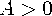c

specifies the nominal per-period coupon rate, expressed as a fraction.

 Range: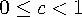n

specifies the number of coupons per period.

 Range: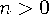and is an integer
K

specifies the number of remaining coupons.

 Range: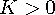and is an integer
k0

specifies the time from the present date to the first coupon date, expressed in terms of the number of periods.

 Range: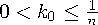y

specifies the nominal per-period yield-to-maturity, expressed as a fraction.

 Range: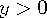The DURP function returns the value
where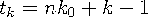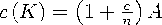and where
```data _null_;
d=durp(1000,1/100,4,14,.33/2,.10);
put d;
run;```

The value returned is 3.26496.Previous Page | Next Page | Top of Page